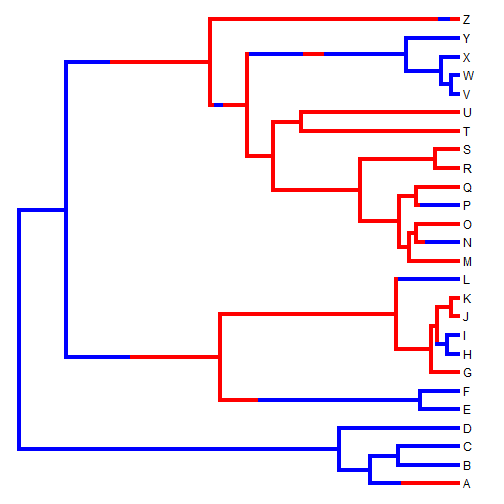## Friday, March 18, 2016

### Fitting a multivariate BM process in which the matrices in different subtrees different by a constant

A phytools user contacted me recently to ask the following question about the function `evol.vcv`, which fits a model in which the VCV matrix of the Brownian process differs in arbitrary ways in different parts of the tree. This could amount to either a different correlation structure, or to different rates of character evolution, or both.

“The method implemented in `evol.vcv` asks a different question [than the one I am interested in], which is whether the evolutionary variance-covariance matrices in the parts of the tree painted by different states are significantly different. I would be very interested in the possibility of modifying the `evol.vcv` method to estimate matrices for the different parts of the tree, but under a proportionality constraint, so that you are then only testing whether the magnitudes of the variance-covariance matrices are different.”

This is not too difficult to imagine. In this case, all we need to do, in fact, is stretch the branches of the tree according to our mapped state, fit a one matrix model to the stretched tree, and optimize the stretching to maximize the likelihood.

Here is a little demo of how this can be done using a tree with two mapped regimes:

``````library(phytools)
tree
``````
``````##
## Phylogenetic tree with 26 tips and 25 internal nodes.
##
## Tip labels:
##  A, B, C, D, E, F, ...
##
## The tree includes a mapped, 2-state discrete character with states:
##  a, b
##
## Rooted; includes branch lengths.
``````
``````plot(tree,lwd=4,colors=setNames(c("blue","red"),letters[1:2]))
````````````X
``````
``````##         [,1]        [,2]
## A  0.3937416  0.25057679
## B -0.1924272 -0.05333178
## C -0.8268161 -0.31647169
## D -1.7806980 -1.03204905
## E -1.3626050  2.04095426
## F -1.2420612  2.95629271
## G -2.1357379  2.60005833
## H -1.8056314  2.94426652
## I -2.2225492  2.90187279
## J -2.2968208  0.99597712
## K -2.5579238  0.94370881
## L -1.4393516  4.76403082
## M -2.7992479  1.01421201
## N -1.3686460  2.00027978
## O -3.8114330 -0.72720413
## P -1.4840348  0.66810845
## Q -2.7595162 -0.09196671
## R -3.8046969 -0.97445030
## S -2.7835255  1.44478295
## T -1.1039130 -3.45626088
## U  1.2860697 -2.66774252
## V -1.4486301 -4.04472576
## W -1.2437545 -3.80878423
## X -1.5335598 -3.93634575
## Y -0.7534220 -3.64595781
## Z -3.5675176 -1.41508111
``````
``````## function to stretch edges
stretch.edges<-function(tree,stretch){
tree\$edge.length<-rowSums(tree\$mapped.edge*
(matrix(1,nrow(tree\$edge),1)%*%
stretch[colnames(tree\$mapped.edge)]))
tree
}
## likelihood function
lik<-function(theta,tree,X){
ff<-setNames(c(1,theta),colnames(tree\$mapped.edge))
print(ff)
obj<-stretch.edges(tree,ff)
obj<-paintSubTree(obj,Ntip(obj)+1,"1")
fit<-evol.vcv(obj,X)
print(fit\$logL1)
-fit\$logL1
}
## optimize
object<-optimize(lik,interval=c(0,1000),tree=tree,X=X)
``````
``````##       a       b
##   1.000 381.966
##  -106.9862
##       a       b
##   1.000 618.034
##  -114.8134
##       a       b
##   1.000 236.068
##  -99.59825
##       a       b
##   1.000 145.898
##  -92.8272
##        a        b
##  1.00000 90.16994
##  -86.87198
##        a        b
##  1.00000 55.72809
##  -81.92101
##        a        b
##  1.00000 34.44185
##  -78.1147
##        a        b
##  1.00000 21.28624
##  -75.51852
##        a        b
##  1.00000 13.15562
##  -74.11245
##        a        b
## 1.000000 8.130619
##  -73.7922
##        a        b
## 1.000000 6.804372
##  -73.91565
##        a        b
## 1.000000 9.352473
##  -73.78256
##        a        b
## 1.000000 8.859582
##  -73.77679
##       a       b
## 1.00000 8.88838
##  -73.7768
##       a       b
## 1.00000 8.87124
##  -73.77679
##        a        b
## 1.000000 8.871362
##  -73.77679
##        a        b
## 1.000000 8.871321
##  -73.77679
##        a        b
## 1.000000 8.871403
##  -73.77679
##        a        b
## 1.000000 8.871362
##  -73.77679
``````
``````object
``````
``````## \$minimum
##  8.871362
##
## \$objective
##  73.77679
``````

This gives us the result of the optimization - the ratio of matrices between each mapped state (the generating ratio was 10) - as well as the log-likelihood.

In this case, we could also compare to the full model, and if we do so, we find that the proportional model is nearly as good:

``````fit<-evol.vcv(tree,X)
fit
``````
``````## ML single-matrix model:
##  R[1,1]  R[1,2]  R[2,2]  k   log(L)
## fitted   2.1107  1.346   4.717   5   -80.6344
##
## ML multi-matrix model:
##  R[1,1]  R[1,2]  R[2,2]  k   log(L)
## a    0.7358  0.3774  0.5421  8   -72.2319
## b    3.561   2.6759  10.2167
##
## P-value (based on X^2): 8e-04
##
## R thinks it has found the ML solution.
``````

Note that this is by no means the most efficient way to code this because it is written using `evol.vcv` internally, which also fits a full model for every iteration of the numerical optimization.

Data for this experiment were simulated as follows:

``````tree<-pbtree(n=26,tip.label=LETTERS,scale=2)
Q<-matrix(c(-1,1,1,-1),2,2)
rownames(Q)<-colnames(Q)<-letters[1:2]
tree<-sim.history(tree,Q)
V<-list(a=matrix(c(1,0.5,0.5,1),2,2),b=matrix(c(10,5,5,10),2,2))
X<-sim.corrs(tree,V)
``````

1.Hi Liam,

I also implemented such an approach in mvMORPH which is maybe slightly more efficient.

Just have to use the following code:

mvBM(tree, X, param=list(constraint="proportional"))

All the best,

Julien

1.Neat. Have you compared it to evol.vcv for real datasets? I should try mvMORPH in a future workshop.
Thanks Julien. All the best, Liam

2.Yes I obtains the same results when fitting multiple independent rates matrices:

## Simulate the data
set.seed(14)
tree<-pbtree(n=26,tip.label=LETTERS,scale=2)
Q<-matrix(c(-1,1,1,-1),2,2)
rownames(Q)<-colnames(Q)<-letters[1:2]
tree<-sim.history(tree,Q)
V<-list(a=matrix(c(1,0.5,0.5,1),2,2),b=matrix(c(10,5,5,10),2,2))
X<-sim.corrs(tree,V)

## Fit:
mvBM(tree,X)
evol.vcv(tree,X)

There is still the possibility to add various "constraints" (shared eigen vectors, shared variances, shared correlations, proportional matrices...) through the "param" list. I will update the package soon... and try to make a clearer vignette.
I heavily rely on your simmap format! Thank again,

All the best,

Julien

Note: due to the very large amount of spam, all comments are now automatically submitted for moderation.